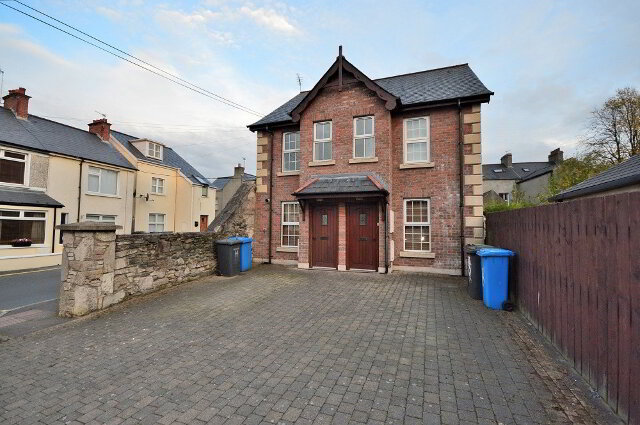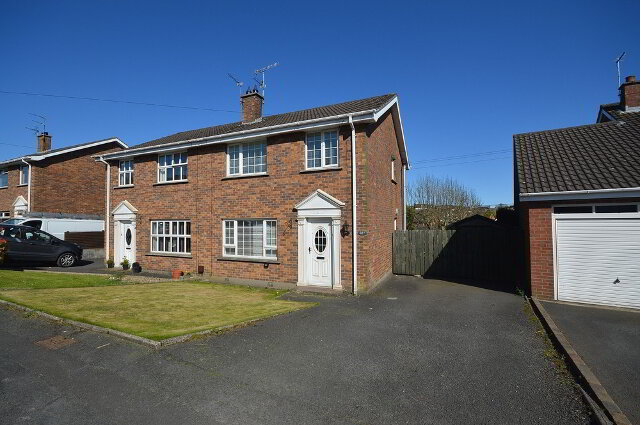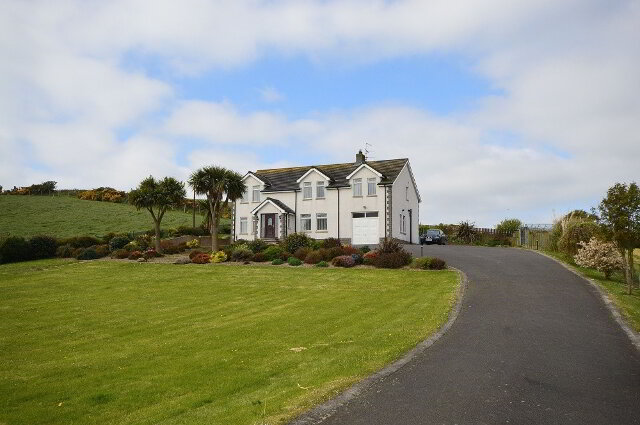Type
Sale/Rent
Min Price
Max Price
Min Beds
Max Beds

# Property For Rent in Ards Peninsula Showing properties 1 to 7 of 7

Sort by
• LetLET
• Let agreedLet agreed £600 / month
• LetLET
• LetLET
• LetLET
• LetLET
• LetLET
• 1